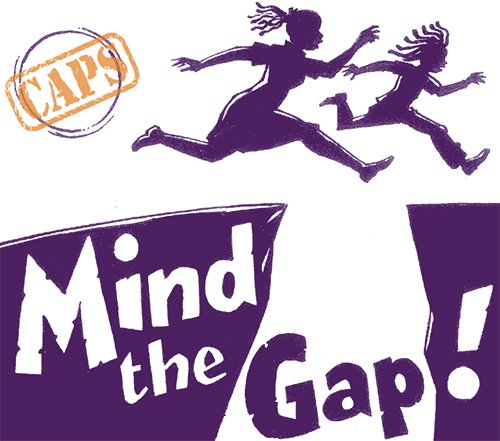# Mathematics Grade 12 Learning Resources for CAPS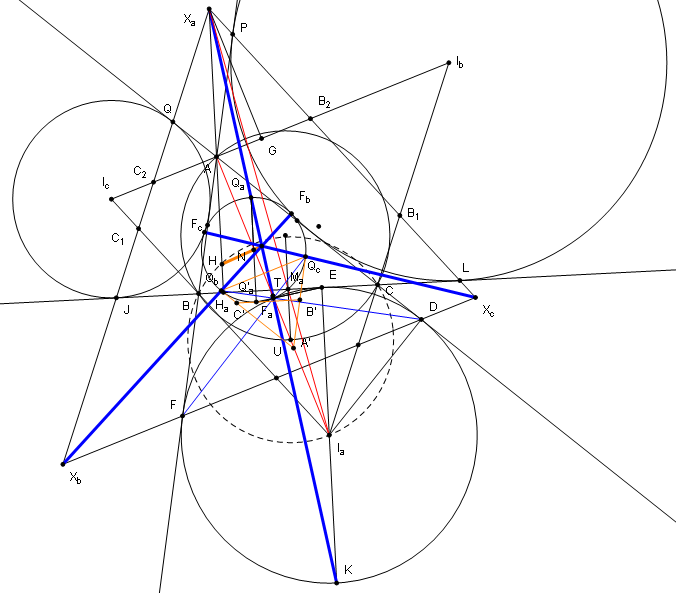Euclidean geometry Grade 12 Questions and Answers:Euclidean geometry is a mathematical system attributed to Alexandrian Greek mathematician Euclid, which he described in his textbook on geometry: the Elements. Euclid’s method consists in assuming a small set of intuitively appealing axioms, and deducing many other propositions from these. Types of triangles  Name Diagram Properties Scalene All sides and angles are different.…#### Grade 12 Mathematics Question Papers and Memos 2020 September

Grade 12 Mathematics Question Papers and Memos 2020 September: About Mathematics Grade 12 About Grade 12 Mathematics: Mathematics is a language that makes use of symbols and notations for describing numerical, geometric and graphical relationships. It is a human activity that involves observing, representing and investigating patterns and qualitative relationships in physical and social phenomena and between mathematical objects themselves.…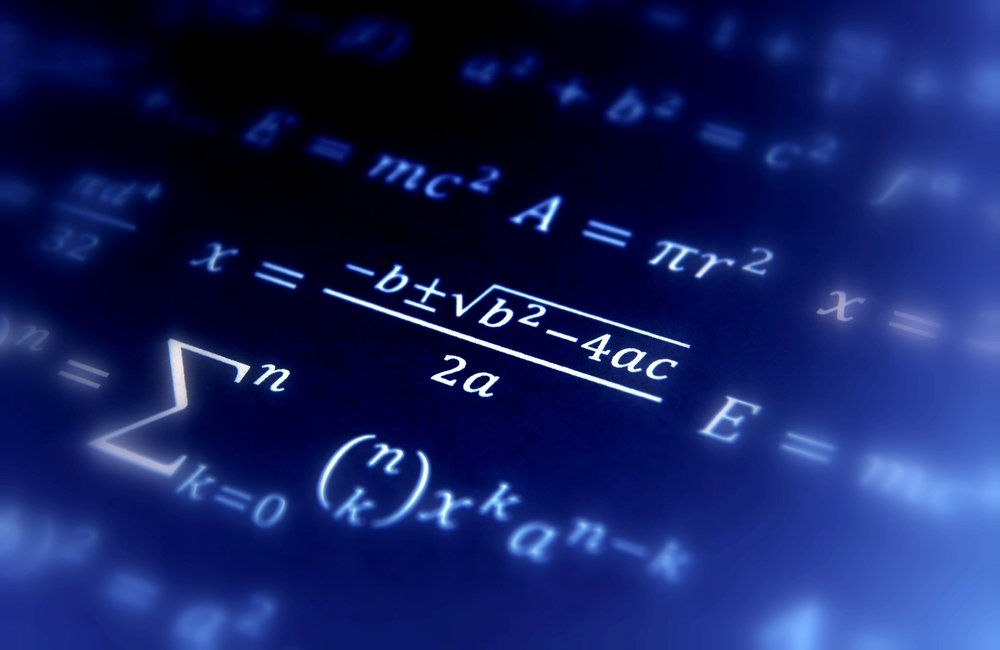#### Mathematics June 2020 Past Exam Papers and Memos

Mathematics Grade 12 June 2020 Exam Papers and Memo About Mathematics Grade 12 About Grade 12 Mathematics: Mathematics is a language that makes use of symbols and notations for describing numerical, geometric and graphical relationships. It is a human activity that involves observing, representing and investigating patterns and qualitative relationships in physical and social phenomena and between mathematical objects themselves.…#### Mathematics Grade 12 March 2020 Exam Papers and Memo

Mathematics Grade 12 March 2020 Exam Papers and Memo About Mathematics Grade 12 About Grade 12 Mathematics: Mathematics is a language that makes use of symbols and notations for describing numerical, geometric and graphical relationships. It is a human activity that involves observing, representing and investigating patterns and qualitative relationships in physical and social phenomena and between mathematical objects themselves.…#### Mathematics Grade 12 Question papers and memorandums

MATHEMATICS GRADE 12 QUESTION PAPERS AND MEMORANDUMS: On this page we have collected mathematics grade 12 question papers for April, June, September, and November with memos. The papers are for 2022, 2021, 2020, 2019 and more. All papers are in pdf downloadable format.  2022 Mathematics Question papers 2021 Mathematics Question papers June 2021 Mathematics Grade 12 2021 Provincial Question Papers…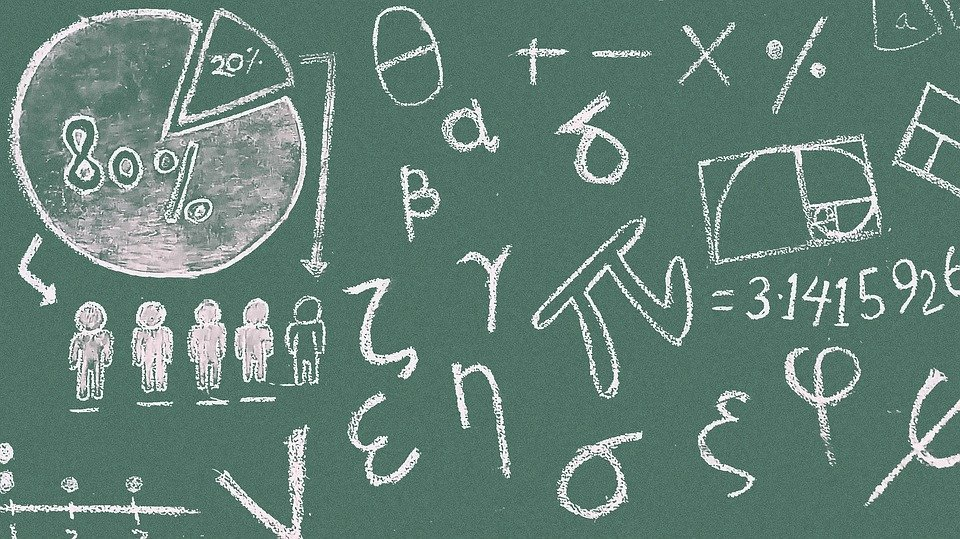#### Grade 12 Mathematics Paper 1 and 2 November 2019 Memorandum pdf

Grade 12 Mathematics Paper 1 and Paper 2 November 2019 Memorandum pdf: About Mathematics Grade 12 About Grade 12 Mathematics: Mathematics is a language that makes use of symbols and notations for describing numerical, geometric and graphical relationships. It is a human activity that involves observing, representing and investigating patterns and qualitative relationships in physical and social phenomena and between…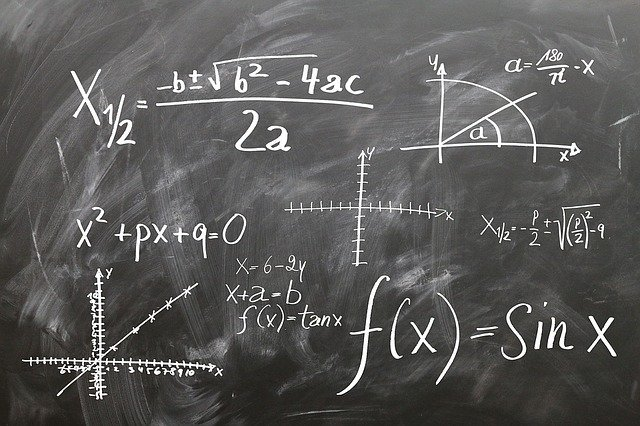#### Grade 12 Mathematics Question Papers and Memos 2019

Download Grade 12 Mathematics Question Papers and Memos for 2019 in Pdf and Word formats: About Mathematics Grade 12 About Grade 12 Mathematics: Mathematics is a language that makes use of symbols and notations for describing numerical, geometric and graphical relationships. It is a human activity that involves observing, representing and investigating patterns and qualitative relationships in physical and social…